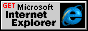# Matrix Filter

Internet Development Index

Resizes, rotates, or reverses the content of the object using matrix transformation.

Syntax

HTML Scripting Internet Explorer 5.5 or later object.style.filter ="progid:DXImageTransform.Microsoft.Matrix(sProperties)" Internet Explorer 5.5 or later

Possible Values

 sProperties String that specifies one or more properties exposed by the filter.

Members Table

AttributePropertyDescription
DxDx Sets or retrieves the X component of the augmenting vector for linear transformations.
DyDy Sets or retrieves the Y component of the augmenting vector for linear transformations.
enabledEnabled Sets or retrieves a value that indicates whether the filter is enabled.
FilterTypeFilterType Sets or retrieves the method used to define pixels of new content.
M11M11 Sets or retrieves the first row/first column matrix entry for linear transformations.
M12M12 Sets or retrieves the first row/second column matrix entry for linear transformations.
M21M21 Sets or retrieves the second row/first column matrix entry for linear transformations.
M22M22 Sets or retrieves the second row/second column matrix entry for linear transformations.
SizingMethodSizingMethod Sets or retrieves a value that indicates whether the container is resized to fit the resulting image.

Remarks

Use the Matrix filter to create the following simple effects.

• Flip horizontal. Negate the M11 and M12 entry values.
• Flip vertical. Negate the M21 and M22 entry values.
• Resize. Multiply each matrix entry by the same factor. The content size changes in proportion to the multiple used. See the following examples.
• Rotate. Adjust the default matrix entries by the appropriate trigonometry function. See the following examples.

These effects are possible through the use of linear transforms. The Matrix filter creates a linear transformation of the displayed content with a 2x2 matrix augmented by a linear vector. Maximizing the effects available with the linear transform matrix requires a thorough understanding of linear algebra. However, for the simple functions described previously, no linear algebra is required.

The final image produced by the Matrix filter is an interpolation of the previous content. Using the padding property minimizes the chance of clipping at the edge of the final image.

The object that the filter is applied to must have layout before the filter effect displays. You can give the object layout by setting the height or width property, setting the position property to absolute, setting the writingMode property to tb-rl, or setting the contentEditable property to true.

You can assign multiple filters or transitions to an object by declaring each in the filter property of the object. The following div declaration assigns two filters and a Wheel transition to a div element.

```<DIV STYLE="width:100%; filter:
progid:DXImageTransform.Microsoft.MotionBlur(strength=13, direction=310)
progid:DXImageTransform.Microsoft.Wheel(duration=3);">
Blurry text with smudge of gray.</div>     ```
When multiple filters are applied to an object, each filter is process in source order, with the exception of procedural surfaces, which are computed first. To emphasize a filter's effect, place it last in source order or on the object's parent. Always place transitions last in source order.

Examples

You can script a resizing function for the Matrix filter by multiplying each matrix entry by a common multiple. The resulting filter increases or decreases the size of the oObj input object and its content by the value of flMultiplier.

```<SCRIPT>
<!-- fnResize function -->
//oObj input requires an matrix filter applied.
//flMultiplier input defines the amount by which the oObj is resized.
function fnResize(oObj,flMultiplier)
{
oObj.filters.item(0).M11 *= flMultiplier;
oObj.filters.item(0).M12 *= flMultiplier;
oObj.filters.item(0).M21 *= flMultiplier;
oObj.filters.item(0).M22 *= flMultiplier;
}
</SCRIPT>
```

You can script a more complex rotating function by applying trigonometry functions to the entries of the Matrix filter. Given an object with a Matrix filter applied, the object is rotated by the angle entered.

```<SCRIPT>
var deg2radians = Math.PI * 2 / 360;
<!-- fnSetRotation function -->
//oObj input requires an matrix filter applied.
//deg input defines the requested angle of rotation.
oObj.filters.item(0).M11 = costheta;
oObj.filters.item(0).M12 = -sintheta;
oObj.filters.item(0).M21 = sintheta;
oObj.filters.item(0).M22 = costheta;
}
</SCRIPT>
```

You can combine the two previous functions and create a simple animation. The animation is driven by the onfilterchange event. The following example spins and expands the text of a div object. You can animate other style-related properties in this manner.

```<SCRIPT><!-- fnSetRotation function -->
//oObj input requires that a matrix filter be applied.
//deg input defines the requested angle of rotation.
var deg2radians = Math.PI * 2 / 360;
function fnSetRotation(oObj, deg)
oObj.filters.item(0).M11 = costheta;
oObj.filters.item(0).M12 = -sintheta;
oObj.filters.item(0).M21 = sintheta;
oObj.filters.item(0).M22 = costheta;
}
<!-- fnResize function -->
//oObj input requires that a matrix filter be applied.
//flMultiplier input defines the amount by which the oObj is resized.
function fnResize(oObj, flMultiplier)
{    oObj.filters.item(0).M11 *= flMultiplier;
oObj.filters.item(0).M12 *= flMultiplier;
oObj.filters.item(0).M21 *= flMultiplier;
oObj.filters.item(0).M22 *= flMultiplier;
}
var iCount = 400;
<!-- fnSpin function -->
function fnSpin(oObj)
{
<!-- The function chosen for flMultiple defines size changes in the animation. -->
var flMultiple = iCount/720;
iCount += 4;
<!-- The number of 360-degree rotations is three. -->
if (iCount>=360*3)     {
oObj.onfilterchange = null;
}
fnSetRotation(oObj, iCount);
fnResize(oObj, flMultiple);
}
</SCRIPT>
<!-- When loaded, the onfilterchange event is fired as the filter makes
its initial settings.  This starts the animation.-->
<DIV ID="oDiv" STYLE="position:absolute;
filter:progid:DXImageTransform.Microsoft.Matrix(sizingMethod='auto expand')"
onfilterchange="fnSpin(this)" >
SOME TEXT<BR/>
SOME TEXT<BR/>
SOME TEXT<BR/>
SOME TEXT<BR/>
</DIV>
</DIV>
```